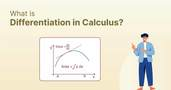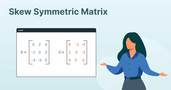Mastering Ratio and Proportion Questions: Tips, Tricks, and Techniques!

# Mastering Ratio and Proportion Questions: Tips, Tricks, and Techniques!

clickHere
Updated on Aug 31, 2023 10:29 IST

A ratio is a numerical comparison of two numbers, but a proportion is an equation stating that two ratios are equal. This article will teach us how to solve the ratio and proportion questions. The article also consists of solved and unsolved questions for learning and practice.

Ratio and proportion are two key mathematical concepts that are used to compare two or more quantities. A ratio is a numerical comparison of two numbers, but a proportion is an equation stating that two ratios are equal.

This tutorial will teach us how to compute ratios and proportions using various problems.

Must Check: Top Math Courses for Data Science

Let us begin with a formal definition of Ratio and Proportion.

## What is Ratio?

The Ratio is a way to compare two quantities by division. In simple terms, it is a way to compare how much of one thing is compared to another thing.

It can be expressed in several ways, using a colon (e.g. 3:4) and using a division (e.g. 3/4). Sometimes the Ratio of two numbers can also be expressed using the word “to”.

Example: You have 7 red balls and 3 blue balls. Then the Ratio of:

• Red balls to Blue balls is 7:3.
• Blue balls to Red Balls is 3:7

Note: Two numbers must have the same unit of measurement.

## What is Proportion?

A Proportion is an equation that states that two ratios are equal. In simple terms, it means that two ratios represent the same relationship between numbers.

It can be written as a fraction set equal to another fraction, such as a/b = c/d.

Example: For a recipe that calls for 2 cups of flour to make 12 cookies. If you want to make 6 cookies, you would use 1 cup of flour.

Here, the proportion will be:

2 cup of floor / 12 cookies = 1 cup of floor / 6 cookies

Until now, you have a clear understanding of what ratio and proportion are.

Now, let’s take one more example of Ratio and proportion together to understand better what Ratios and Proportions are and how to calculate them.

### Example of Ratio and Proportion

#### You are making lemonade using a recipe that involves that require following ingredients:

Lemon = 4

Cup of Sugar = 1

Liters of Water = 2

Then the Ratio of lemon to sugar to water is 4:1:2, i.e., for every 4 lemons, you are using 1 cup of sugar and 2 litres of water.

Now, you decided to make lemonade of 12 lemons, so how much water and sugar do you need?

Here, the concept of proportion comes into action. Here, we will set up a proportion based on the original recipe.

For Lemons

• Original Ratio = 4 lemon
• New Quantity = 12 lemons

For Sugar

• Original Ratio = 1 cup of sugar
• New Quantity = x cup of sugar

For Water

• Original Ratio = 2 litres of water
• New Quantity = y litre of water

Now, using the proportion of lemon to calculate the proportion of water and sugar:

(4 lemons/1 cup of sugar) = (12 lemons/x cups of sugar)

Now, cross-multiplying, we get:

4*x = 12*1

=> x = 3

So, you will 3 cups of sugar for 12 lemons.

Similarly, you can calculate the proportion of water for 12 lemons.

(4 lemons/2 litres of water) = 12 lemons/y litres of water)

Cross-multiplying, we get:

4*y = 12*2

=> y = 6

So, you will need 6 litre of water to make lemonade of 12 lemons.

Hence, to make lemonade of 12 lemons, you have to add 3 cups of sugar and 6 litres of water to maintain the same taste as the original.

## Ratio and Proportion Questions

#### 1. Divide 50 into two people in a ratio of 3:2.

Answer: Let’s think of a question like you have to divide 50 into 5 equal parts to two people: the first person will take 3 parts of 50, and the second person will take the remaining 2 parts of 50.

therefore,

First Person = (3/5) * 50 = 30

Second Person = (2/5) * 50 = 20

#### 2. Are 2:3 and 4:6 equivalent ratios?

Answer: Yes, because 2/3 = 4/6 (simplifying the numerator and denominator, 4/6 = 2*2 / 2*3 = 2/3).

#### 3. If 5 books cost INR 100, how much do 8 book cost?

Cost of 5 books = 100

=> Cost of 1 book = 100/5 = 20

So, the cost of 8 books = 8*20 = 160

or

Let the cost of 8 books will be INR x

then,

100/ 5 = x/8 (Cost of n book/ n number of books)

cross-multiplying both side, we get

100*8 = 5*x

=> x = 100*8/5 = 160

=> x = 160

Hence, the cost of 8 books will be INR 160.

#### 4. The ratio of ages of A and B is 4:5. If the age of A is 24, then calculate the age of B.

Answer: Here, we will use the same concept we used in the above question.

Let the age of B is x, then

4/5 = 24/x

=> x = (24*5)/4 = 30

Hence, the age of B is 30 years.

#### 5. In what ratio should water be mixed with juice to get a mixture containing 75% juice?

Answer: Let the ratio of water that should be mixed with the juice to get the mixture containing juice is x:1.

Therefore,

(x/x+1)*100 = 75

=> x/x+1 = 3/4

=> x = 3

hence, the ratio is 3:1.

Till now, you have a clear understanding of how to calculate the ratio and proportion. So, let’s have some ratio and proportion questions for practice.

A) 15, 25
B) 9, 15
C) 12, 20
D) 3, 5

A) 23:33:60
B) 3:3:6
C) 23:30:60
D) 10:10:20

A) 78%
B) 80%
C) 82%
D) 85%

A) 50
B) 100
C) 150
D) 200

A) 0.2
B) 0.5
C) 0.05
D) 5

## Conclusion

A ratio is a numerical comparison of two numbers, but a proportion is an equation stating that two ratios are equal.

In this article, we have discussed how to solve the ratio and proportion questions. The article also consists of solved and unsolved questions for learning and practice.

Hope you will like the article.

Keep Learning!!

Keep Sharing!!Difference Between Distance and Displacement
Confused with the difference between distance and displacement. Don’t worry; this article will briefly define distance, displacement, and the difference between them.Scalars and Vectors: Understanding the Key Differences
Physical quantity with magnitude and no direction is known as a scalar quantity and a physical quantity with magnitude and directions is known as a vector quantity. In this article,...read moreHow Vectors are Used in Machine Learning
Vectors are mathematical objects that contain both magnitude and direction, and they can be represented by the directed line segments (lines having directions) whose lengths are their magnitude. It is...read moreDifferential Calculus for Data Science
Differentiation is the process of finding the derivative of a function. The derivative of a function measures the rate of change of a function. In this article, we will discuss...read moreUnderstanding Set Theory – What, Where, Why, and How do we use it in Data Science
Set in mathematics is a well-defined collection of objects that doesn’t vary from person to person. In this article, we will briefly discuss set theory, its representation, subset, cardinality, union...read moreCross Product of two Vectors
Cross product is a binary operation (multiplication) that is performed on two vectors, and the resultant vector is perpendicular to both the given vectors. In this article, we will discuss...read moreTranspose of a Matrix
Transpose of a matrix is a matrix flipped over its main diagonal, switching the matrix’s rows and column indices. In this article, we will briefly discuss what transpose of a...read moreIn Machine Learning Algorithms, we use distance metrics such as Euclidean, Manhattan, Minkowski, and Hamming.
In this article, we will briefly discuss one such metric, i.e., Manhattan Distance.A square matrix is said to be a skew-symmetric matrix if it is equal to the negative of its transpose. This article, we will briefly discuss skew-symmetric matrix, properties and...read more

## FAQs

What is Ratio?

A ratio is a relationship between two numbers, showing how many times one value contains or is contained within the other. It's a way to compare two quantities.

What is Propotion?

A Proportion is an equation that states that two ratios are equal. In simple terms, it means that two ratios represent the same relationship between numbers.

How is a proportion different from a ratio?

While a ratio is a way to compare two quantities, a proportion is an equation that states that two ratios are equal.

clickHere

Vikram has a Postgraduate degree in Applied Mathematics, with a keen interest in Data Science and Machine Learning. He has experience of 2+ years in content creation in Mathematics, Statistics, Data Science, and Mac... Read Full Bio

## Trending Data Science CoursesBecome a Data Science Professional with IIT Certification in Advanced ProgrammingBecome a Data Science Professional with IIT Certif...
Guvi5.0AI For Everyone
Coursera4.5Machine Learning
Coursera4.6Postgraduate Program in Data Science with Generative AIPostgraduate Program in Data Science with Generati...
Praxis Tech School4.8Starts 5 DecBecome a Data Science Professional with IIT Certification in Advanced ProgrammingBecome a Data Science Professional with IIT Certif...
Guvi5.0Starts 15 Dec

## Top Picks & New Arrivals